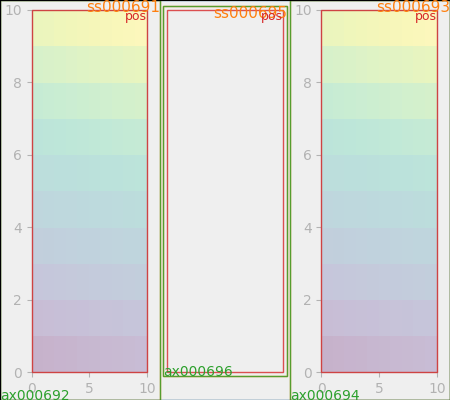# Constrained Layout Guide¶

How to use constrained-layout to fit plots within your figure cleanly.

constrained_layout automatically adjusts subplots and decorations like legends and colorbars so that they fit in the figure window while still preserving, as best they can, the logical layout requested by the user.

constrained_layout is similar to tight_layout, but uses a constraint solver to determine the size of axes that allows them to fit.

constrained_layout needs to be activated before any axes are added to a figure. Two ways of doing so are

Those are described in detail throughout the following sections.

Warning

Currently Constrained Layout is experimental. The behaviour and API are subject to change, or the whole functionality may be removed without a deprecation period. If you require your plots to be absolutely reproducible, get the Axes positions after running Constrained Layout and use ax.set_position() in your code with constrained_layout=False.

## Simple Example¶

In Matplotlib, the location of axes (including subplots) are specified in normalized figure coordinates. It can happen that your axis labels or titles (or sometimes even ticklabels) go outside the figure area, and are thus clipped.

# sphinx_gallery_thumbnail_number = 18

import matplotlib.pyplot as plt
import matplotlib.colors as mcolors
import matplotlib.gridspec as gridspec
import numpy as np

plt.rcParams['savefig.facecolor'] = "0.8"
plt.rcParams['figure.figsize'] = 4.5, 4.

def example_plot(ax, fontsize=12, nodec=False):
ax.plot([1, 2])

ax.locator_params(nbins=3)
if not nodec:
ax.set_xlabel('x-label', fontsize=fontsize)
ax.set_ylabel('y-label', fontsize=fontsize)
ax.set_title('Title', fontsize=fontsize)
else:
ax.set_xticklabels('')
ax.set_yticklabels('')

fig, ax = plt.subplots(constrained_layout=False)
example_plot(ax, fontsize=24)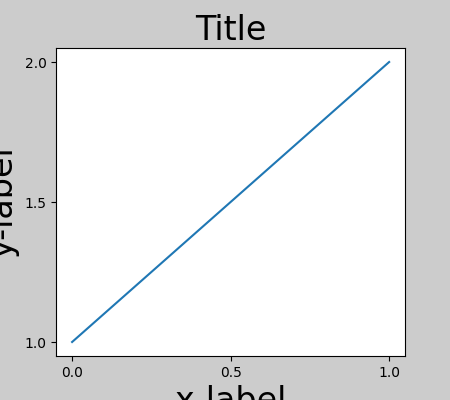To prevent this, the location of axes needs to be adjusted. For subplots, this can be done by adjusting the subplot params (Move the edge of an axes to make room for tick labels). However, specifying your figure with the constrained_layout=True kwarg will do the adjusting automatically.

fig, ax = plt.subplots(constrained_layout=True)
example_plot(ax, fontsize=24)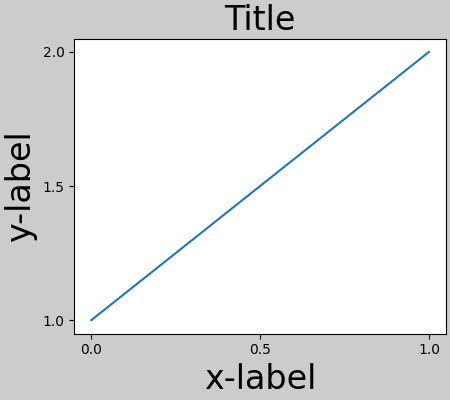When you have multiple subplots, often you see labels of different axes overlapping each other.

fig, axs = plt.subplots(2, 2, constrained_layout=False)
for ax in axs.flat:
example_plot(ax)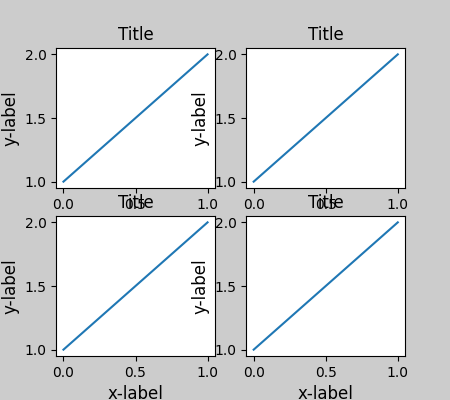Specifying constrained_layout=True in the call to plt.subplots causes the layout to be properly constrained.

fig, axs = plt.subplots(2, 2, constrained_layout=True)
for ax in axs.flat:
example_plot(ax)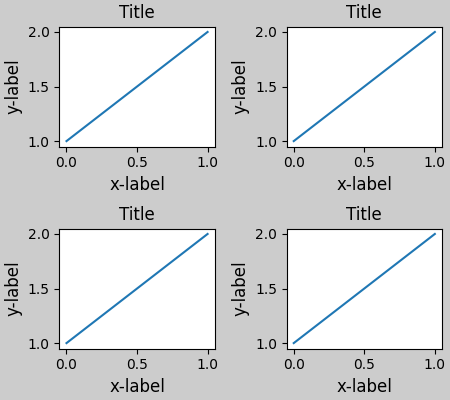## Colorbars¶

If you create a colorbar with the colorbar() command you need to make room for it. constrained_layout does this automatically. Note that if you specify use_gridspec=True it will be ignored because this option is made for improving the layout via tight_layout.

Note

For the pcolormesh kwargs (pc_kwargs) we use a dictionary. Below we will assign one colorbar to a number of axes each containing a ScalarMappable; specifying the norm and colormap ensures the colorbar is accurate for all the axes.

arr = np.arange(100).reshape((10, 10))
norm = mcolors.Normalize(vmin=0., vmax=100.)
# see note above: this makes all pcolormesh calls consistent:
pc_kwargs = {'rasterized': True, 'cmap': 'viridis', 'norm': norm}
fig, ax = plt.subplots(figsize=(4, 4), constrained_layout=True)
im = ax.pcolormesh(arr, **pc_kwargs)
fig.colorbar(im, ax=ax, shrink=0.6)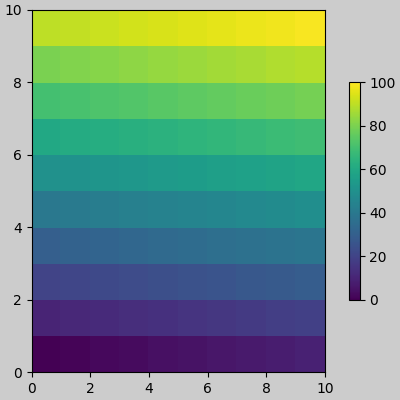Out:

<matplotlib.colorbar.Colorbar object at 0x7f155a07f460>


If you specify a list of axes (or other iterable container) to the ax argument of colorbar, constrained_layout will take space from the specified axes.

fig, axs = plt.subplots(2, 2, figsize=(4, 4), constrained_layout=True)
for ax in axs.flat:
im = ax.pcolormesh(arr, **pc_kwargs)
fig.colorbar(im, ax=axs, shrink=0.6)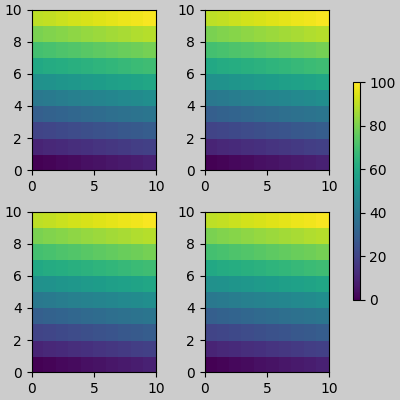Out:

<matplotlib.colorbar.Colorbar object at 0x7f152883fa00>


If you specify a list of axes from inside a grid of axes, the colorbar will steal space appropriately, and leave a gap, but all subplots will still be the same size.

fig, axs = plt.subplots(3, 3, figsize=(4, 4), constrained_layout=True)
for ax in axs.flat:
im = ax.pcolormesh(arr, **pc_kwargs)
fig.colorbar(im, ax=axs[1:, ][:, 1], shrink=0.8)
fig.colorbar(im, ax=axs[:, -1], shrink=0.6)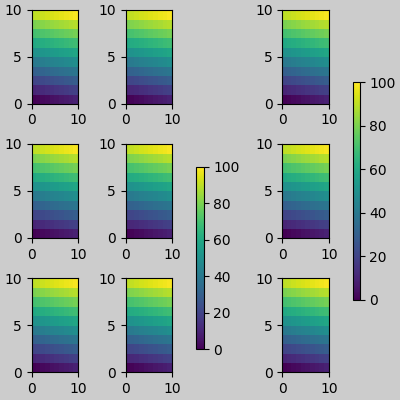Out:

<matplotlib.colorbar.Colorbar object at 0x7f15289c0190>


Note that there is a bit of a subtlety when specifying a single axes as the parent. In the following, it might be desirable and expected for the colorbars to line up, but they don't because the colorbar paired with the bottom axes is tied to the subplotspec of the axes, and hence shrinks when the gridspec-level colorbar is added.

fig, axs = plt.subplots(3, 1, figsize=(4, 4), constrained_layout=True)
for ax in axs[:2]:
im = ax.pcolormesh(arr, **pc_kwargs)
fig.colorbar(im, ax=axs[:2], shrink=0.6)
im = axs.pcolormesh(arr, **pc_kwargs)
fig.colorbar(im, ax=axs, shrink=0.6)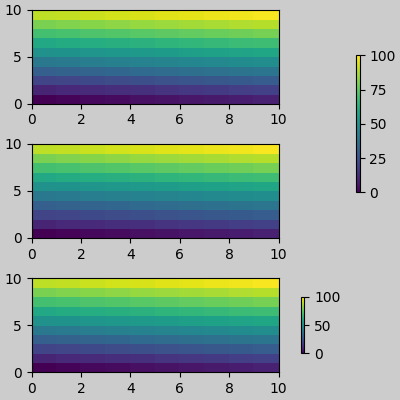Out:

<matplotlib.colorbar.Colorbar object at 0x7f1528211400>


The API to make a single-axes behave like a list of axes is to specify it as a list (or other iterable container), as below:

fig, axs = plt.subplots(3, 1, figsize=(4, 4), constrained_layout=True)
for ax in axs[:2]:
im = ax.pcolormesh(arr, **pc_kwargs)
fig.colorbar(im, ax=axs[:2], shrink=0.6)
im = axs.pcolormesh(arr, **pc_kwargs)
fig.colorbar(im, ax=[axs], shrink=0.6)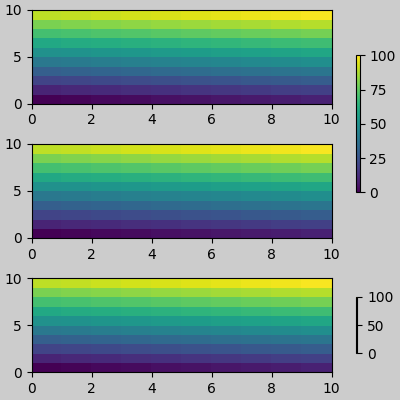Out:

<matplotlib.colorbar.Colorbar object at 0x7f1527e2d220>


## Suptitle¶

constrained_layout can also make room for suptitle.

fig, axs = plt.subplots(2, 2, figsize=(4, 4), constrained_layout=True)
for ax in axs.flat:
im = ax.pcolormesh(arr, **pc_kwargs)
fig.colorbar(im, ax=axs, shrink=0.6)
fig.suptitle('Big Suptitle')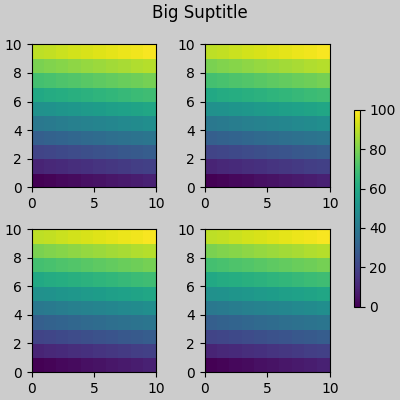Out:

Text(0.5, 0.98, 'Big Suptitle')


## Legends¶

Legends can be placed outside of their parent axis. Constrained-layout is designed to handle this for Axes.legend(). However, constrained-layout does not handle legends being created via Figure.legend() (yet).

fig, ax = plt.subplots(constrained_layout=True)
ax.plot(np.arange(10), label='This is a plot')
ax.legend(loc='center left', bbox_to_anchor=(0.8, 0.5))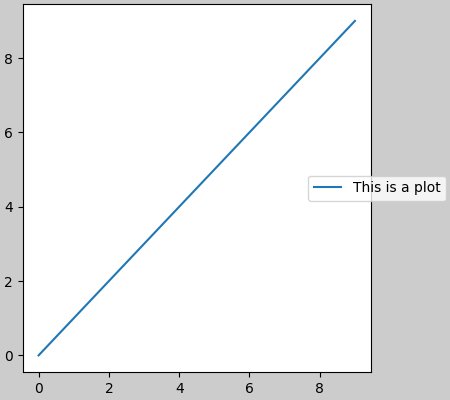Out:

<matplotlib.legend.Legend object at 0x7f152811a2e0>


However, this will steal space from a subplot layout:

fig, axs = plt.subplots(1, 2, figsize=(4, 2), constrained_layout=True)
axs.plot(np.arange(10))
axs.plot(np.arange(10), label='This is a plot')
axs.legend(loc='center left', bbox_to_anchor=(0.8, 0.5))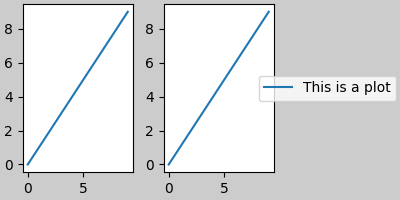Out:

<matplotlib.legend.Legend object at 0x7f1526ab3310>


In order for a legend or other artist to not steal space from the subplot layout, we can leg.set_in_layout(False). Of course this can mean the legend ends up cropped, but can be useful if the plot is subsequently called with fig.savefig('outname.png', bbox_inches='tight'). Note, however, that the legend's get_in_layout status will have to be toggled again to make the saved file work, and we must manually trigger a draw if we want constrained_layout to adjust the size of the axes before printing.

fig, axs = plt.subplots(1, 2, figsize=(4, 2), constrained_layout=True)

axs.plot(np.arange(10))
axs.plot(np.arange(10), label='This is a plot')
leg = axs.legend(loc='center left', bbox_to_anchor=(0.8, 0.5))
leg.set_in_layout(False)
# trigger a draw so that constrained_layout is executed once
# before we turn it off when printing....
fig.canvas.draw()
# we want the legend included in the bbox_inches='tight' calcs.
leg.set_in_layout(True)
# we don't want the layout to change at this point.
fig.set_constrained_layout(False)
fig.savefig('CL01.png', bbox_inches='tight', dpi=100)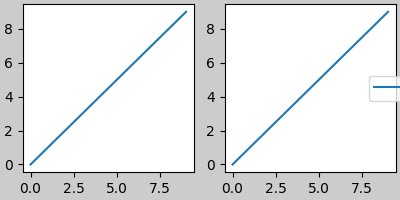The saved file looks like: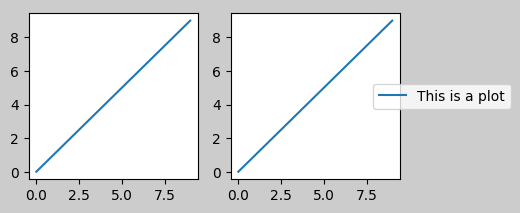A better way to get around this awkwardness is to simply use the legend method provided by Figure.legend:

fig, axs = plt.subplots(1, 2, figsize=(4, 2), constrained_layout=True)
axs.plot(np.arange(10))
lines = axs.plot(np.arange(10), label='This is a plot')
labels = [l.get_label() for l in lines]
leg = fig.legend(lines, labels, loc='center left',
bbox_to_anchor=(0.8, 0.5), bbox_transform=axs.transAxes)
fig.savefig('CL02.png', bbox_inches='tight', dpi=100)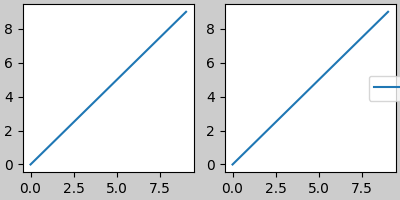The saved file looks like: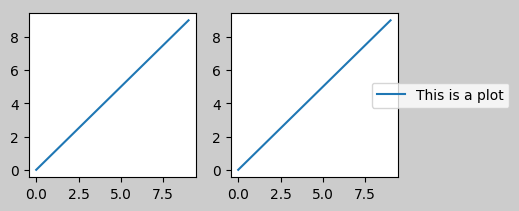For constrained_layout, we have implemented a padding around the edge of each axes. This padding sets the distance from the edge of the plot, and the minimum distance between adjacent plots. It is specified in inches by the keyword arguments w_pad and h_pad to the function set_constrained_layout_pads:

fig, axs = plt.subplots(2, 2, constrained_layout=True)
for ax in axs.flat:
example_plot(ax, nodec=True)
ax.set_xticklabels('')
ax.set_yticklabels('')
hspace=0., wspace=0.)

fig, axs = plt.subplots(2, 2, constrained_layout=True)
for ax in axs.flat:
example_plot(ax, nodec=True)
ax.set_xticklabels('')
ax.set_yticklabels('')
hspace=0., wspace=0.)

•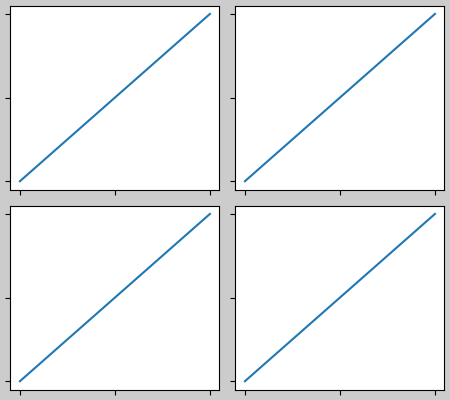•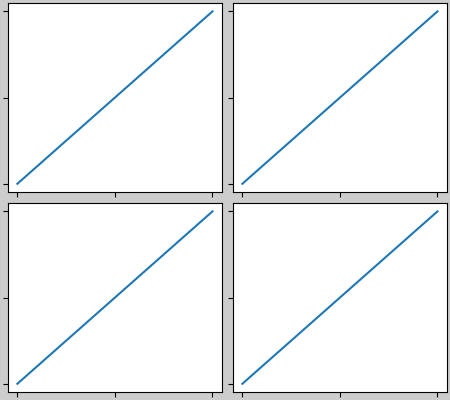Spacing between subplots is set by wspace and hspace. There are specified as a fraction of the size of the subplot group as a whole. If the size of the figure is changed, then these spaces change in proportion. Note in the below how the space at the edges doesn't change from the above, but the space between subplots does.

fig, axs = plt.subplots(2, 2, constrained_layout=True)
for ax in axs.flat:
example_plot(ax, nodec=True)
ax.set_xticklabels('')
ax.set_yticklabels('')
hspace=0.2, wspace=0.2)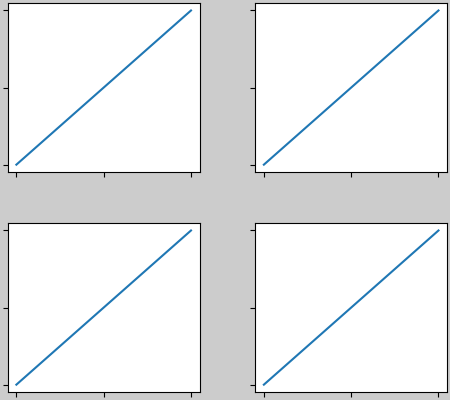### Spacing with colorbars¶

Colorbars will be placed wspace and hsapce apart from other subplots. The padding between the colorbar and the axis it is attached to will never be less than w_pad (for a vertical colorbar) or h_pad (for a horizontal colorbar). Note the use of the pad kwarg here in the colorbar call. It defaults to 0.02 of the size of the axis it is attached to.

fig, axs = plt.subplots(2, 2, constrained_layout=True)
for ax in axs.flat:
pc = ax.pcolormesh(arr, **pc_kwargs)
ax.set_xticklabels('')
ax.set_yticklabels('')
hspace=0.2, wspace=0.2)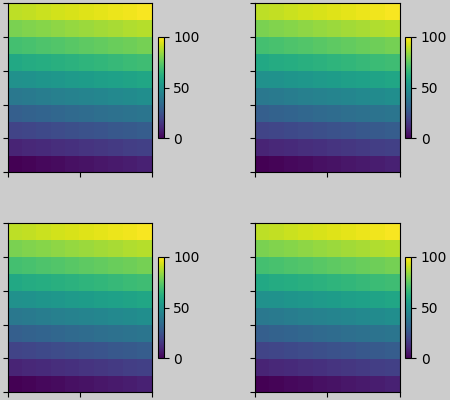In the above example, the colorbar will not ever be closer than 2 pts to the plot, but if we want it a bit further away, we can specify its value for pad to be non-zero.

fig, axs = plt.subplots(2, 2, constrained_layout=True)
for ax in axs.flat:
pc = ax.pcolormesh(arr, **pc_kwargs)
ax.set_xticklabels('')
ax.set_yticklabels('')
hspace=0.2, wspace=0.2)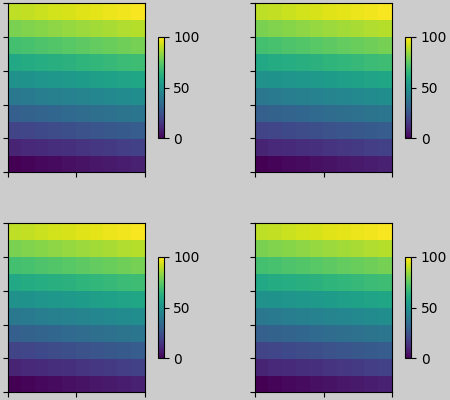## rcParams¶

There are five rcParams that can be set, either in a script or in the matplotlibrc file. They all have the prefix figure.constrained_layout:

• use: Whether to use constrained_layout. Default is False
• w_pad, h_pad: Padding around axes objects.
Float representing inches. Default is 3./72. inches (3 pts)
• wspace, hspace: Space between subplot groups.
Float representing a fraction of the subplot widths being separated. Default is 0.02.
plt.rcParams['figure.constrained_layout.use'] = True
fig, axs = plt.subplots(2, 2, figsize=(3, 3))
for ax in axs.flat:
example_plot(ax)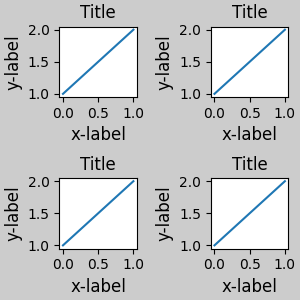## Use with GridSpec¶

constrained_layout is meant to be used with subplots() or GridSpec() and add_subplot().

Note that in what follows constrained_layout=True

fig = plt.figure()

gs1 = gridspec.GridSpec(2, 1, figure=fig)

example_plot(ax1)
example_plot(ax2)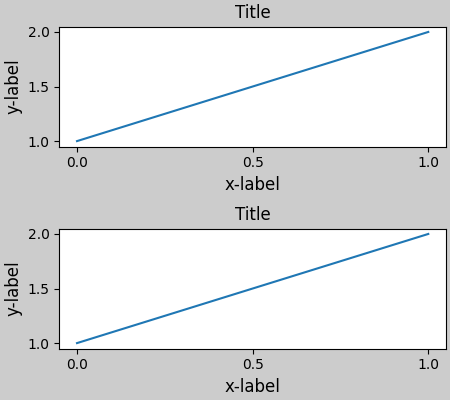More complicated gridspec layouts are possible. Note here we use the convenience functions add_gridspec and subgridspec.

fig = plt.figure()

gs1 = gs0.subgridspec(2, 1)

example_plot(ax1)
example_plot(ax2)

gs2 = gs0.subgridspec(3, 1)

for ss in gs2:
example_plot(ax)
ax.set_title("")
ax.set_xlabel("")

ax.set_xlabel("x-label", fontsize=12)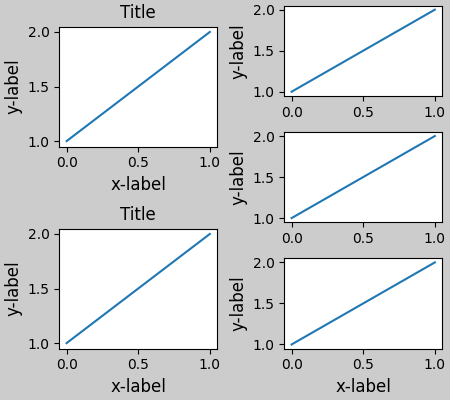Out:

Text(0.5, 0, 'x-label')


Note that in the above the left and columns don't have the same vertical extent. If we want the top and bottom of the two grids to line up then they need to be in the same gridspec:

fig = plt.figure()

example_plot(ax1)
example_plot(ax2)

example_plot(ax)
example_plot(ax)
example_plot(ax)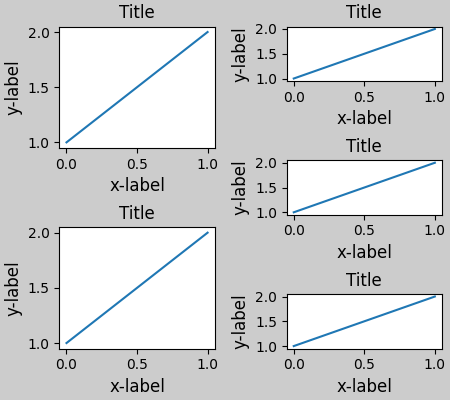This example uses two gridspecs to have the colorbar only pertain to one set of pcolors. Note how the left column is wider than the two right-hand columns because of this. Of course, if you wanted the subplots to be the same size you only needed one gridspec.

def docomplicated(suptitle=None):
fig = plt.figure()
gs0 = fig.add_gridspec(1, 2, figure=fig, width_ratios=[1., 2.])
gsl = gs0.subgridspec(2, 1)
gsr = gs0.subgridspec(2, 2)

for gs in gsl:
example_plot(ax)
axs = []
for gs in gsr:
pcm = ax.pcolormesh(arr, **pc_kwargs)
ax.set_xlabel('x-label')
ax.set_ylabel('y-label')
ax.set_title('title')

axs += [ax]
fig.colorbar(pcm, ax=axs)
if suptitle is not None:
fig.suptitle(suptitle)

docomplicated()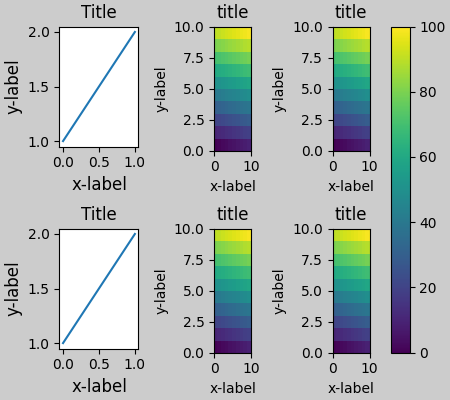## Manually setting axes positions¶

There can be good reasons to manually set an axes position. A manual call to set_position will set the axes so constrained_layout has no effect on it anymore. (Note that constrained_layout still leaves the space for the axes that is moved).

fig, axs = plt.subplots(1, 2)
example_plot(axs, fontsize=12)
axs.set_position([0.2, 0.2, 0.4, 0.4])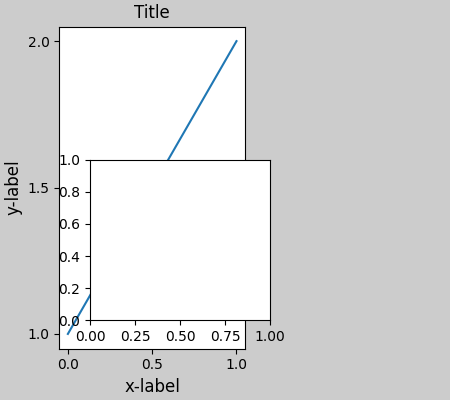If you want an inset axes in data-space, you need to manually execute the layout using fig.execute_constrained_layout() call. The inset figure will then be properly positioned. However, it will not be properly positioned if the size of the figure is subsequently changed. Similarly, if the figure is printed to another backend, there may be slight changes of location due to small differences in how the backends render fonts.

from matplotlib.transforms import Bbox

fig, axs = plt.subplots(1, 2)
example_plot(axs, fontsize=12)
fig.execute_constrained_layout()
# put into data-space:
bb_data_ax2 = Bbox.from_bounds(0.5, 1., 0.2, 0.4)
disp_coords = axs.transData.transform(bb_data_ax2)
fig_coords_ax2 = fig.transFigure.inverted().transform(disp_coords)
bb_ax2 = Bbox(fig_coords_ax2)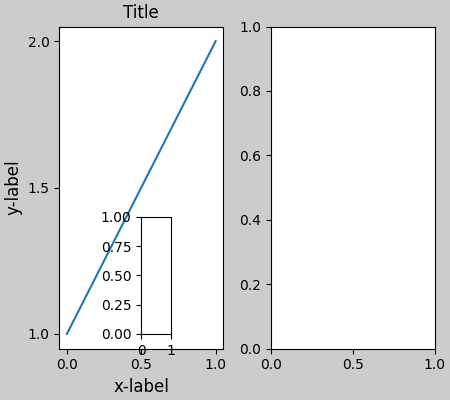## Manually turning off constrained_layout¶

constrained_layout usually adjusts the axes positions on each draw of the figure. If you want to get the spacing provided by constrained_layout but not have it update, then do the initial draw and then call fig.set_constrained_layout(False). This is potentially useful for animations where the tick labels may change length.

Note that constrained_layout is turned off for ZOOM and PAN GUI events for the backends that use the toolbar. This prevents the axes from changing position during zooming and panning.

## Limitations¶

### Incompatible functions¶

constrained_layout will not work on subplots created via the pyplot.subplot command. The reason is that each of these commands creates a separate GridSpec instance and constrained_layout uses (nested) gridspecs to carry out the layout. So the following fails to yield a nice layout:

fig = plt.figure()

ax1 = plt.subplot(221)
ax2 = plt.subplot(223)
ax3 = plt.subplot(122)

example_plot(ax1)
example_plot(ax2)
example_plot(ax3)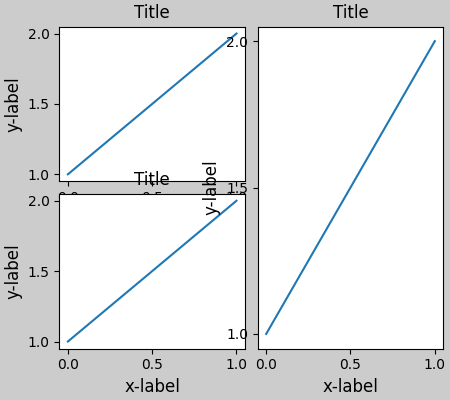Of course that layout is possible using a gridspec:

fig = plt.figure()

example_plot(ax1)
example_plot(ax2)
example_plot(ax3)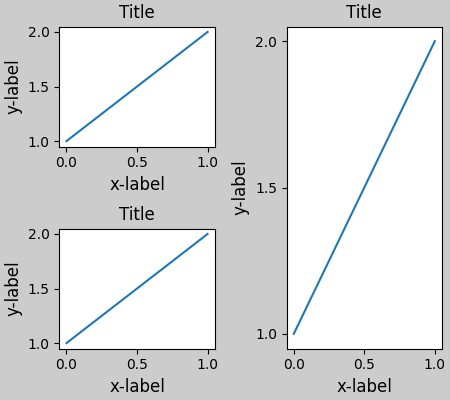Similarly, subplot2grid() doesn't work for the same reason: each call creates a different parent gridspec.

fig = plt.figure()

ax1 = plt.subplot2grid((3, 3), (0, 0))
ax2 = plt.subplot2grid((3, 3), (0, 1), colspan=2)
ax3 = plt.subplot2grid((3, 3), (1, 0), colspan=2, rowspan=2)
ax4 = plt.subplot2grid((3, 3), (1, 2), rowspan=2)

example_plot(ax1)
example_plot(ax2)
example_plot(ax3)
example_plot(ax4)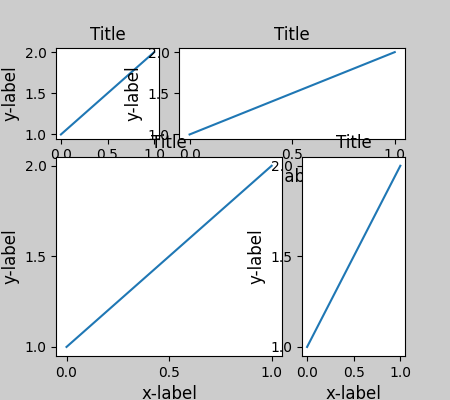The way to make this plot compatible with constrained_layout is again to use gridspec directly

fig = plt.figure()

example_plot(ax1)
example_plot(ax2)
example_plot(ax3)
example_plot(ax4)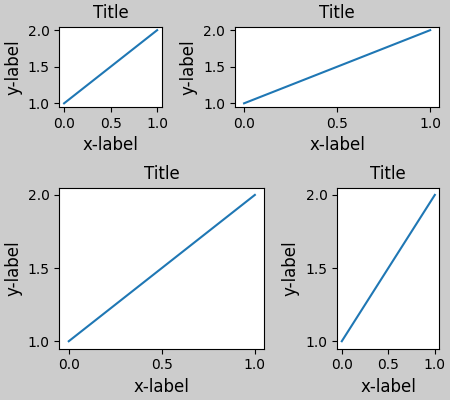### Other Caveats¶

• constrained_layout only considers ticklabels, axis labels, titles, and legends. Thus, other artists may be clipped and also may overlap.
• It assumes that the extra space needed for ticklabels, axis labels, and titles is independent of original location of axes. This is often true, but there are rare cases where it is not.
• There are small differences in how the backends handle rendering fonts, so the results will not be pixel-identical.
• An artist using axes coordinates that extend beyond the axes boundary will result in unusual layouts when added to an axes. This can be avoided by adding the artist directly to the Figure using add_artist(). See ConnectionPatch for an example.

## Debugging¶

Constrained-layout can fail in somewhat unexpected ways. Because it uses a constraint solver the solver can find solutions that are mathematically correct, but that aren't at all what the user wants. The usual failure mode is for all sizes to collapse to their smallest allowable value. If this happens, it is for one of two reasons:

1. There was not enough room for the elements you were requesting to draw.
2. There is a bug - in which case open an issue at https://github.com/matplotlib/matplotlib/issues.

If there is a bug, please report with a self-contained example that does not require outside data or dependencies (other than numpy).

## Notes on the algorithm¶

The algorithm for the constraint is relatively straightforward, but has some complexity due to the complex ways we can layout a figure.

### Figure layout¶

Figures are laid out in a hierarchy:

1. Figure: fig = plt.figure()
1. Gridspec gs0 = gridspec.GridSpec(1, 2, figure=fig)

1. Subplotspec: ss = gs[0, 0]
1. Axes: ax0 = fig.add_subplot(ss)
2. Subplotspec: ss = gs[0, 1]
1. Gridspec: gsR = gridspec.GridSpecFromSubplotSpec(2, 1, ss)

• Subplotspec: ss = gsR[0, 0]

• Axes: axR0 = fig.add_subplot(ss)
• Subplotspec: ss = gsR[1, 0]

• Axes: axR1 = fig.add_subplot(ss)

Each item has a layoutbox associated with it. The nesting of gridspecs created with GridSpecFromSubplotSpec can be arbitrarily deep.

Each Axes has two layoutboxes. The first one, ax._layoutbox represents the outside of the Axes and all its decorations (i.e. ticklabels, axis labels, etc.). The second layoutbox corresponds to the Axes' ax.position, which sets where in the figure the spines are placed.

Why so many stacked containers? Ideally, all that would be needed are the Axes layout boxes. For the Gridspec case, a container is needed if the Gridspec is nested via GridSpecFromSubplotSpec. At the top level, it is desirable for symmetry, but it also makes room for suptitle.

For the Subplotspec/Axes case, Axes often have colorbars or other annotations that need to be packaged inside the Subplotspec, hence the need for the outer layer.

### Simple case: one Axes¶

For a single Axes the layout is straight forward. The Figure and outer Gridspec layoutboxes coincide. The Subplotspec and Axes boxes also coincide because the Axes has no colorbar. Note the difference between the red pos box and the green ax box is set by the size of the decorations around the Axes.

In the code, this is accomplished by the entries in do_constrained_layout() like:

ax._poslayoutbox.edit_left_margin_min(-bbox.x0 + pos.x0 + w_padt)

from matplotlib._layoutbox import plot_children

fig, ax = plt.subplots(constrained_layout=True)
example_plot(ax, fontsize=24)
plot_children(fig, fig._layoutbox, printit=False)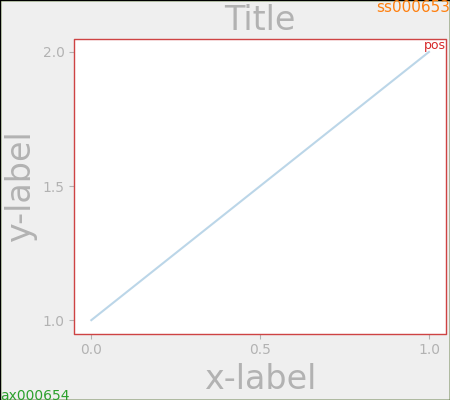### Simple case: two Axes¶

For this case, the Axes layoutboxes and the Subplotspec boxes still co-incide. However, because the decorations in the right-hand plot are so much smaller than the left-hand, so the right-hand layoutboxes are smaller.

The Subplotspec boxes are laid out in the code in the subroutine arange_subplotspecs(), which simply checks the subplotspecs in the code against one another and stacks them appropriately.

The two pos axes are lined up. Because they have the same minimum row, they are lined up at the top. Because they have the same maximum row they are lined up at the bottom. In the code this is accomplished via the calls to layoutbox.align. If there was more than one row, then the same horizontal alignment would occur between the rows.

The two pos axes are given the same width because the subplotspecs occupy the same number of columns. This is accomplished in the code where dcols0 is compared to dcolsC. If they are equal, then their widths are constrained to be equal.

While it is a bit subtle in this case, note that the division between the Subplotspecs is not centered, but has been moved to the right to make space for the larger labels on the left-hand plot.

fig, ax = plt.subplots(1, 2, constrained_layout=True)
example_plot(ax, fontsize=32)
example_plot(ax, fontsize=8)
plot_children(fig, fig._layoutbox, printit=False)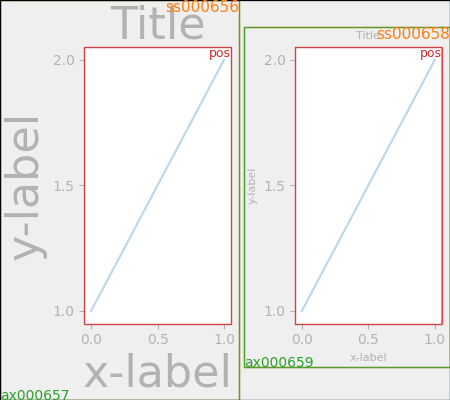### Two Axes and colorbar¶

Adding a colorbar makes it clear why the Subplotspec layoutboxes must be different from the axes layoutboxes. Here we see the left-hand subplotspec has more room to accommodate the colorbar, and that there are two green ax boxes inside the ss box.

Note that the width of the pos boxes is still the same because of the constraint on their widths because their subplotspecs occupy the same number of columns (one in this example).

The colorbar layout logic is contained in make_axes which calls _constrained_layout.layoutcolorbarsingle() for cbars attached to a single axes, and _constrained_layout.layoutcolorbargridspec() if the colorbar is associated with a gridspec.

fig, ax = plt.subplots(1, 2, constrained_layout=True)
im = ax.pcolormesh(arr, **pc_kwargs)
fig.colorbar(im, ax=ax, shrink=0.6)
im = ax.pcolormesh(arr, **pc_kwargs)
plot_children(fig, fig._layoutbox, printit=False)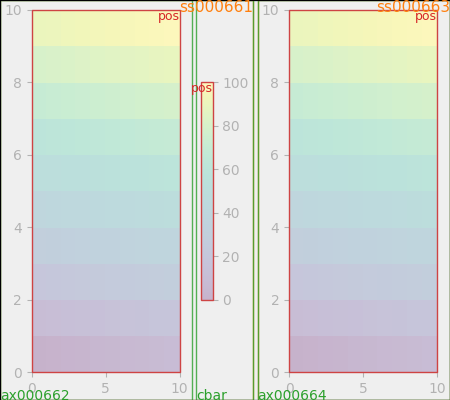### Colorbar associated with a Gridspec¶

This example shows the Subplotspec layoutboxes being made smaller by a colorbar layoutbox. The size of the colorbar layoutbox is set to be shrink smaller than the vertical extent of the pos layoutboxes in the gridspec, and it is made to be centered between those two points.

fig, axs = plt.subplots(2, 2, constrained_layout=True)
for ax in axs.flat:
im = ax.pcolormesh(arr, **pc_kwargs)
fig.colorbar(im, ax=axs, shrink=0.6)
plot_children(fig, fig._layoutbox, printit=False)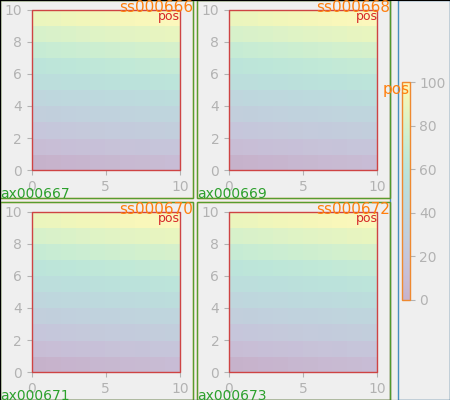### Uneven sized Axes¶

There are two ways to make axes have an uneven size in a Gridspec layout, either by specifying them to cross Gridspecs rows or columns, or by specifying width and height ratios.

The first method is used here. The constraint that makes the heights be correct is in the code where drowsC < drows0 which in this case would be 1 is less than 2. So we constrain the height of the 1-row Axes to be less than half the height of the 2-row Axes.

Note

This algorithm can be wrong if the decorations attached to the smaller axes are very large, so there is an unaccounted-for edge case.

fig = plt.figure(constrained_layout=True)
gs = gridspec.GridSpec(2, 2, figure=fig)
im = ax.pcolormesh(arr, **pc_kwargs)
im = ax.pcolormesh(arr, **pc_kwargs)
im = ax.pcolormesh(arr, **pc_kwargs)
plot_children(fig, fig._layoutbox, printit=False)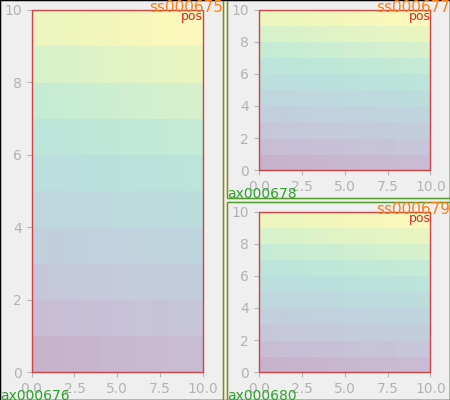Height and width ratios are accommodated with the same part of the code with the smaller axes always constrained to be less in size than the larger.

fig = plt.figure(constrained_layout=True)
gs = gridspec.GridSpec(3, 2, figure=fig,
height_ratios=[1., 0.5, 1.5],
width_ratios=[1.2, 0.8])
im = ax.pcolormesh(arr, **pc_kwargs)
im = ax.pcolormesh(arr, **pc_kwargs)
im = ax.pcolormesh(arr, **pc_kwargs)
im = ax.pcolormesh(arr, **pc_kwargs)
plot_children(fig, fig._layoutbox, printit=False)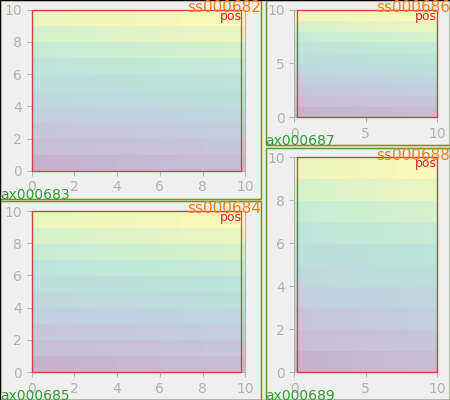### Empty gridspec slots¶

The final piece of the code that has not been explained is what happens if there is an empty gridspec opening. In that case a fake invisible axes is added and we proceed as before. The empty gridspec has no decorations, but the axes position in made the same size as the occupied Axes positions.

This is done at the start of _constrained_layout.do_constrained_layout() (hassubplotspec).

fig = plt.figure(constrained_layout=True)
gs = gridspec.GridSpec(1, 3, figure=fig)
im = ax.pcolormesh(arr, **pc_kwargs)